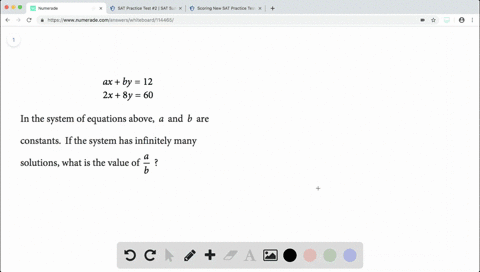Enroll in one of our FREE online STEM summer camps. Space is limited so join now!View Summer Courses### $$\begin{array}{l}{a x+b y=12} \\ {2 x+8 y=60}\en… 01:20Johns Hopkins University Need more help? Fill out this quick form to get professional live tutoring. Get live tutoring Problem 19 An architect wants to use the riser-tread formula to design a stairway with a total rise of 9 feet, a riser height between 7 and 8 inches, and an odd number of steps. With the architect's constraints, which of the following must be the tread depth, in inches, of the stairway? ( 1 foot = 12 inches)$$\begin{array}{l}{\text { A) } 7.2} \\ {\text { B) } 9.5} \\ {\text { C) } 10.6} \\ {\text { D) } 15}\end{array}

Check back soon!
SAT
SAT Practice Test # 6

## Discussion

You must be signed in to discuss.

## Video Transcript

Okay, So for this one, it says with the architects constraints which the falling must be the tread depth and interest. So ultimately, we're looking for the trenta. Okay, So first of all, the things that I have to notice is that they give us a conversion rate, which means that we have to use it. So then we know that one foot is equal to twelve inches and were given the total rise to a height of nine feet. I'm going to go ahead and convert the nine feet interest, and the way I'm going to do that is I'm about to take the nine feet and used to measure announces and say one foot is twelve inches. And the way the reason we want to do it this way so that this feat and this feat canceled out. We're just left with interest on top, which is exactly what we want so that we do that we got a hundred and eight inches is the total rights or height. Then if we have the hundred and eight inches and says that we want to bring it down between seven and eight inches, so we either want little heights stares at her like this where it's seven and just or we want bigger ones of eight inches that will probably give us less. So what's going on? Try to divide that because if we divided the one of eight by seven and by eight will tell us how how many steps will actually get thanks them for doing away inches divided by seven and one eight interest divided by eight. So we divide that by sudden that gives us fifteen point four, and then for the one of eight eight, we got thirteen point five, But then we know that we want an odd number of steps. It says right here on number of steps. So this one rounds up to fourteen, whereas this one round down to fifteen. So we're going to go ahead and choose this one. So now we know that the exact number of steps that we want is fifteen. So the reason why we did this part so that we can actually see how, between how many steps that we're gonna want using these parameters, So it's gonna be between seven and eight inches and then So we got fifteen. So then, if we take that one and divide that by fifteen i'LL tell us so we did a total riser How divided by the number of steps. So give us how much each step will be in terms of the height. So then if we do that went away to find my fifteen gives a seven point two, which works because that is between seventy interests. So each step is going to be seven point two inches high. But again, we're not looking for the height. We're looking for the tread depth. So then we have to take the seven point two inches and convert it using our riser tried formula, which is to age plus tea is equal to twenty five. So I'm gonna put that in for the H, which is two times seven point two plus De is equal to twenty five on then. So I'm just gonna take that twenty five and subtract the two times seven quite too, which is going to give me that he is equal to ten point six inches